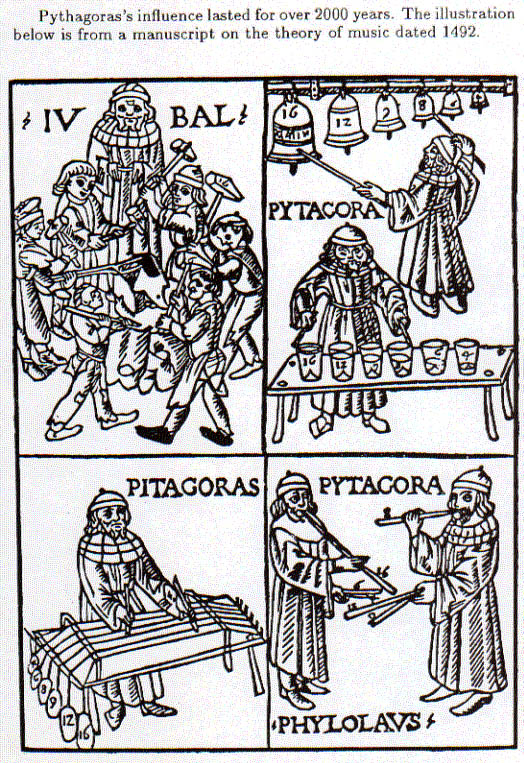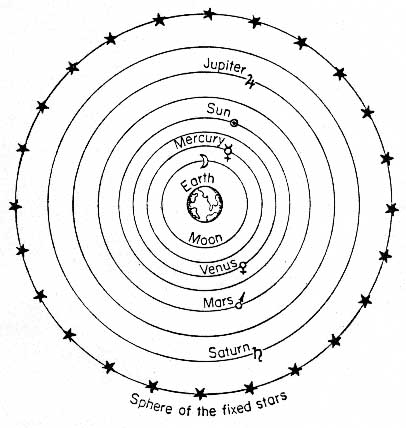3.7 Music of the Spheres and the Lessons of Pythagoras

CONCLUSIONS SO FAR

At this point, we have covered a number of topics and some history of physics, so it is important to step back and understand where we are before going on.

I. Using simple mathematics, Pythagoras was able to describe the basis of almost all musical scales, including the pentatonic, the Western, the chromatic and the Arabic scales. This shows the power and excitement of science. For the first time, Pythagoras could answer the question, WHY? Why are these notes and scales special? The answer is that they are formed in a simple, systematic, and mathematical manner. Most importantly, Pythagoras showed that the notes are not random or arbitrary and that they could be understood on a deeper level.

II. Pythagoras’s discoveries bring up a deeper ‘psychology’ question: scales were first developed by ear: we – and the Neanderthals – choose these particular notes before there was any understanding of mathematics or physics. The notes were chosen simply because they were pleasing to the ear. But, as it turns out, the scales also follow basic mathematical constructs. So the question is, what does this say about our likes and emotions? Is there a mathematical/physical basis to them, as well?

III. The power of spectroscopy. What Pythagoras did was look a physical system (the musical scale), found characteristic frequencies (pitches/notes) and found simple mathematical relationships between the frequencies (ratios of 3/2, for example). This process actually became a fundamental part of physics, and modern physics, in particular.

Modern physics started in the early 1900’s with the concepts of quantum mechanics and relativity. However, the concept of quantum mechanics came about through a process much like Pythagoras’s. The physicist, Neils Bohr, considered a physical system (the hydrogen atom), examined the frequencies characteristic of hydrogen (i.e. the colors of light that a hydrogen lamp produced), and found simple relationships between the frequencies (such as ratios of 32/42).

This process, used by Pythagoras, Bohr and many others, is called spectroscopy (loosely, the study of frequencies) and is at the heart of most modern science. To better understand it, spectroscopy also forms basis of most of the labs in the course. Indeed, strings and air columns fall into the same process:

 Physical system Musical scale Hydrogen atom Air column Frequencies Notes of scale Colors of light Resonances Relationship n/m n2/m2 n× f

MANY CAVEATS TO THIS SCIENTIFIC SUCCESS.

Although Pythagoras ushered in a whole new way of looking at the world, there was still much more to be done in the development of modern science. So, it is also important to understand the limitations up to this point.

1. Pythagoras got lucky: Pythagoras did not actually study the frequencies that made up pleasing intervals and the musical scale. Rather, he just made observations about the lengths of the strings that made intervals and scales. However, it just so happens that the frequency of a string is simple related (i.e. inversely proportional) to the length of the string. So, all the conclusions he reached about ratios of lengths of strings for different intervals, also applies to the ratios of the frequencies in the intervals.

The problem is that this is not true for the tension in the string, for example. In the labs, you use an apparatus like this to study the vibrations of a string where you can change the length or the tension on the string.Pythagoras might have tried to find relationships between the weights needed to form pleasing intervals. However, if you double the weight the frequency changes by Ö 2 not 2, so the ratios for the intervals would not be as simple. Pythagoras might not have figured out what was going on if he used the weights.

Also, the frequency is not simply related to the length when it comes to vibrating bars, like you find on a xylophone or a marimba (the frequency is inversely proportional to the square of the length). If Pythagoras had studied a xylophone, instead of a lyre, things, again, might have turned out quite differently!

Although these possibilities may seem a bit far-fetched, they are not as can be seen from a famous woodcut made in the Middle Ages to explain the theories of Pythagoras:The purpose of the wood cut was to illustrate the Pythagorean ratios and how they applied to musical instruments. The lower right panel shows flutes whose lengths correspond to the Pythagorean ratios. This works out because, like a string, the frequency of an air column (e.g. a flute) is simply related to its length. However, the panel on the lower left has a problem. As discussed above, the weights on the ends of the strings will change the tension in the strings. But, the frequency of the strings is not simply related to the tension, and the strings will not sound according to the Pythagorean intervals. The examples in the upper panels are even more complicated, but suffice it to say, the bells, water glasses, and anvils will not produce the correct intervals.

One of the defining features of the Middle Ages was a reverence for the knowledge passed down from the ancient Greek philosophers. This knowledge was then applied to situations where it did not apply, but there was also no tradition of actually trying something out to see if it was correct. Had anyone bothered to build any of the instruments (except for the flute) in the ratios prescribed in the woodcut, they would have found that the intervals were all wrong.

A crucial step for the development of modern science was the willingness to test the theories of other to see if they really were correct or not. In fact, it was Vincenzo Galilei, Galileo Galilie’s father, who first noticed that there was something wrong with the woodcut. This period marked the beginning of the Renaissance, and we will hear more about Vincenzo later. However, he certainly set the stage for his son to come along and challenge the most basic beliefs about the Universe at the time.

II. While Pythagoras was making lost of progress in mathematics, geometry and music, the Greek astronomers of the time were not doing quite so well. They realized that there were certain "fixed" stars – stars whose relative position in the sky did not change through the seasons. They also noted that there were "wanderers" or planets (planet is the Greek word for wanderer). These planets moved around relative to the background stars.

To explain these observations, the astronomers figured that the fixed stars were attached to a large black sphere that defined the edge of the universe. The planets had to be attached to moving spheres, with each planet on its own sphere. However, these spheres could not be black, otherwise, one could not see through to the stars in back. So, the spheres that the planets were attached to had to be crystal spheres. The "planets" that the astronomers knew about were: the moon, Mercury, Venus, the sun, Mars, Jupiter, and Saturn. Seven, in all, so there were seven crystal spheres. The big question was: why seven? To the Greeks, the answer was obvious: Pythagoras just had his big breakthrough that mathematics could explain phenomena in nature and he now understood why there were seven notes in the musical scale. Thus, there must be the same reason for why there are seven crystal spheres. The astronomers were so convinced of this that they called it the Music of the Spheres. This concept was so powerful that it led astronomy astray for 1500 years!Even many great physicists were taken in by this vision that universe had an order that was musical in nature. The astronomer Kepler became obsessed with trying to fit the orbits of the planets to a musical scale!

This, again, demonstrates the danger of taking knowledge from one field and applying to other fields where it is not appropriate or applicable.

However, as an inspirational force, music did aid in the development of physics long after the planetary orbits were understood. Most of the founders of Q.M. started out with studying music. Strangely enough, the motion of the electron in a hydrogen atom actually does follow the Music of the Spheres, to a certain extent.

III. Just because Pythagoras found a very simple way of constructing the musical scale, it does not mean that it is the only way, or even the simplest way. In fact, there are many different ways of constructing the scale and the Pythagorean way is almost never used.

For example, a piano is tuned on the basis that two half steps should equal one whole step. In the Pythagorean scale a half step = 256/243. So, two half steps gives (256/243)2 = 1.110, while the whole step is 9/8 = 1.125. These are close, but you can hear the difference. One a piano, whole step = 1.122, just in between.

So, just because Pythagoras found a simple mathematical answer, it may not be the whole answer or even the only answer.

IV. There are many things that Pythagoras did not explain. Why is the frequency inversely proportional to the length? Why use the length at all for his studies? Why do we like these intervals? However, Pythagoras did make a great start.